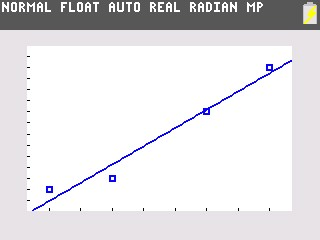# Knowledge Base

## Solution 34506: Calculating and Graphing a Linear Regression on the TI-84 Plus family of graphing calculators.

### How do I calculate and graph a linear regression on the TI-84 Plus family of graphing calculators?

The following example will demonstrate how to calculate a linear regression.

First, you will need to enter the data:

• Press [STAT]  to enter the Stat List Editor.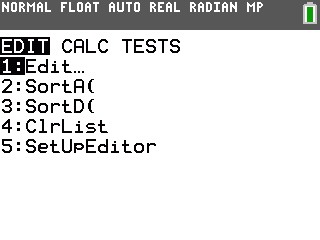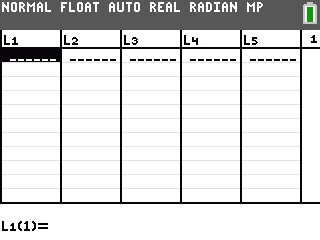• Enter the data {3,5,8,10} in the L1 list and {7,8,14,18} in the L2 list, pressing [ENTER] after each entry to move down along the list.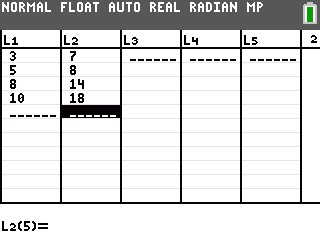To calculate the Linear Regression (ax+b):

• Press [STAT] to enter the statistics menu.• Press the right arrow key to reach the CALC menu and then press 4: LinReg(ax+b).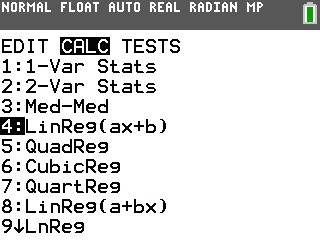• Ensure Xlist is set at L1, Ylist is set at L2 and Store RegEQ is set at Y1 by pressing [VARS] [→] 1:Function and 1:Y1.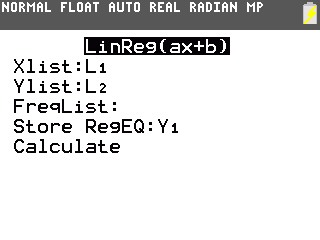• Scroll down to Calculate and press [ENTER].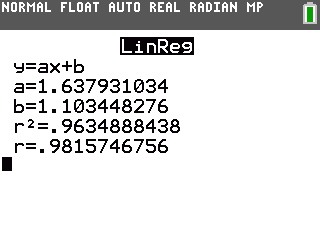The graphing calculator will display the form of the equation as (y=a+bx) and list the values for the two coefficients (a and b). It will store the regression equation to your Y1 function. The correlation coefficient (r and r^2) will be displayed if the diagnostics are on. Note: To turn the diagnostics on, from the home screen, press [2ND] [CATALOG]. Then in the catalog press [ALPHA] [D] to go down to the "D's". Scroll down through the list and select the instruction DiagnosticOn, and press [ENTER] twice and the display should show DONE.

In order to graph the data:

• Press [2ND] [STAT PLOT] to enter the stat plot menu.
• Press  to enter plot 1.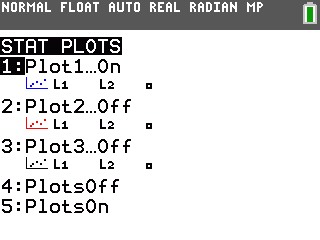• Highlight ON and press [ENTER] to turn the stat plot on and be sure that Xlist is L1 and Ylist is L2.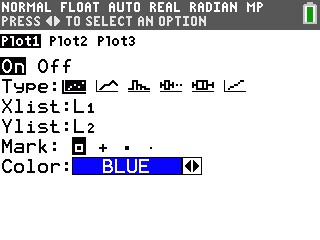• Press [GRAPH].
• Press [ZOOM]  to select ZoomStat.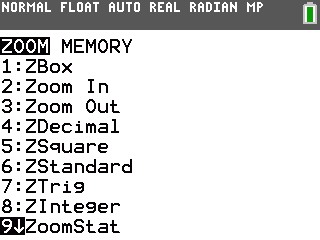• Your graph screen will display the following: